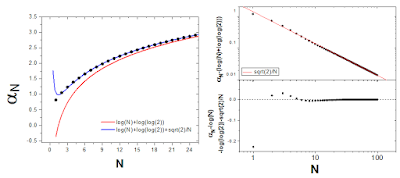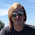## Monday, 10 October 2016

### The Nested Logarithm Constants

Depending on your philosophical interpretation of mathematics, I have either discovered or invented a new number: the Nested Logarithm Constant.

$\alpha$=log(1+log(2+log(3+log(4+log(5+....)))))=0.820359862208789788....

As is natural, the logarithms are base-e. I first calculated this number while reading about the nested radical constant, and I wanted to see if something similar existed for logarithms...and it did! While I cannot be certain that others have not examined this number previously, googling the first few digits only yields random lists of digits. I have entered its decimal expansion into the Online Encyclopedia of Integer Sequences, the closest thing that exists to a Wiki of mildly interesting numbers.

It converges quite rapidly with respect to the final digit, being effectively exact after about 5. The plot below shows the convergence with respect to the final digit, and the fractional deviation from the asymptotic value, with an exponential decay shown for comparison.

While it seems obvious empirically that this converges, it is not proven [by me, see update below]. As far as I can tell, a proof of the convergence of the nested radical constant is also lacking. I would suspect that the nested logarithm constant is both irrational and transcendental, and there is a short proof that the logs of integers are irrational, but I'm not aware of a proof that nested logarithms are irrational.

There are a few extensions one can examine. Changing the base of the logarithm changes the value of the final number, decreasing with increasing base towards zero, and towards infinity with decreasing base.

Another extension is to include an exponent on the integers, such that the number becomes:

$\alpha_{N}=\log(1+\log(2^{N}+\log(3^{N}+...)))$

When N is large, 2$^N$ will be much larger than log(3$^N$+...), so we can take the N out of the exponent and see that $\alpha_{N}$ approaches log(1+N·log(2))  log(N)+log(log(2)). The difference between $\alpha_{N}$ and its large-N approximation decreases inversely with N, with a prefactor empirically* very close to the square root of two. With this reciprocal correction, I can almost write down a closed form expression for $\alpha_{N}$, except it starts to diverge close to N=1, and I don't know if it's a coincidence or not (where does the square root come from?). I would be interested in exploring this further to try to find a closed form expression for the 0.82...constant.The nested logarithm constants as a function of the exponent, and the deviation of two approximations.
There is one more related number of interest. I can calculate log(1+2·log(1+3·log(1+4·log...)))) which converges to roughly 1.663.... I have not investigated this in as much detail, but it is empirically very close to the Somos Quadratic Recurrence Constant, which can be calculated as √(1√(2√(3√(4...)))) and converges to 1.662. Unless I'm lead to believe otherwise I will assume that this is a coincidence.

This investigation started with me playing around with logarithms and seeing what came out, and lead to a few findings that I think are kind of interesting. Maybe when I have the time and energy I'll investigate this further and be able to determine if the nested logarithm constant has an exact value. There is a journal called Experimental Mathematics, which is basically what this is, so perhaps I can send it there.

UPDATE: A commenter on reddit, SpeakKindly, has put forward a simple proof of convergence by establishing an upper bound using induction. I will repeat it here for the readers:

First, consider the related term log(a+log(a+1+log(a+2+log...(log(a+k)))) for some k and a>1. SpeakKindly proved this is less than a for all k. The base case, for k=0, we have no nested terms and just have log(a), which is less than a. Then we assume this is true for k-1 nestings, so we have log(a+log(a+1+log(a+2+log...(log(a+k)))) < log(a+(a+1))=log(2a+1), which is always less than a. The nested logarithm constant is the log(1+(the above term for a=2)), which is less than log(1+2), thus the nested logarithm constant is less than log(3).

Another user, babeltoothe, used a different argument using the integral definition of the logarithm, where the upper limit of each integral was another integral.

*Best power-law fit is 1.004√2 for the prefactor and -0.998 for the exponent.

1.It does converge quite fast.

The number of bits after N iterations is roughly $.02N^2+2.24N-8.5$

This has a correlation coefficient of 1.0 through 5 datapoints.

So, this constant is quite easy to compute lots of digits.

I used this: http://hastebin.com/jubemuxifu.cpp to compute the first 100000 bits.

1.Thanks!
What do you mean by bits in this context?

2.Bits are binary digits.
Like how we have digits that can be 0, 1, 2, 3, . . . 9

The binary equivalent are called bits (each 0 or 1).

So we might say that $1242$ (base 10) has 4 digits, and we would say that $110101$ (base 2) has 6 bits.

Each digit is roughly $log_(2)(10) \approx 3.3219280$ bits.

3.I know what bits are but I'm unsure how it relates to the convergence? Are you talking about the number of converged binary digits in each step after N iterations?

4.Yes.

The quadratic function of $N$ I posted tells how many bits are accurate after N iterations

2.This comment has been removed by the author.

3.I removed my other comment, because this one only has the digits, not the whole output.

Here are the first 15,000 digits (generated from 50,000 bits).

http://hastebin.com/fecaboqese.css

1.Damn that's a lot of digits.

2.This took about 2 minutes on my machine.

60,000 digits would take about twice as long (because the amount required grows by roughly $\sqrt{N}$